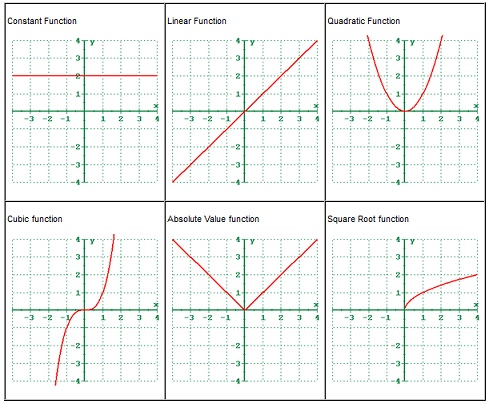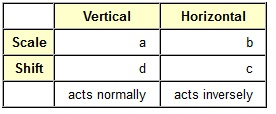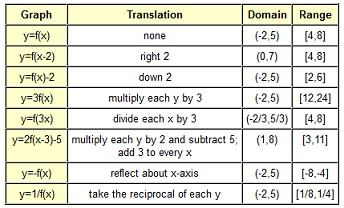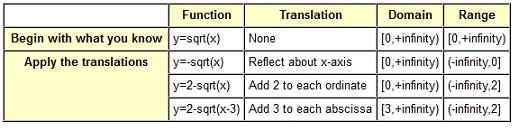#### Theory of Shifting, Reflecting and Stretching Graphs

Basic Definitions of Shifting, Reflecting and Stretching Graphs:

Abscissa: It is the x-coordinate.

Ordinate: It is the y-coordinate.

Shift: It is a translation in which the shape and size of a graph of a function is not modified, however the position of the graph is.

Scale: It is a translation in which the shape and size of the graph of a function is modified.

Reflection: It is a translation in which the graph of a function is mirrored about the axis.

Common Functions:

The part of beauty of mathematics is that nearly everything builds on something else, and if you can understand the foundations, then you can apply latest elements to old. This is the ability that makes comprehension of mathematics possible. When you were to memorize each and every piece of mathematics presented to you devoid of making the connection to other parts, you will:

• Become frustrated at math and
• Not really understand math.

There are few fundamental graphs which we have seen before. By applying translations to such basic graphs, we are capable to get new graphs which still encompass all the properties of the old ones. By understanding the fundamental graphs and the way translations apply to them, we will identify each new graph as a small variation in an old one, not as a wholly different graph which we have never seen before. Understanding such translations will permit us to rapidly recognize and sketch a new function devoid of having to resort to plotting points.

These given below are the common functions we must know the graphs of at this time:

Constant Function: y = c

Linear Function: y = x

Quadratic Function: y = x2

Cubic Function: y = x3

Absolute Value Function: y = |x|

Square Root Function: y = sqrt(x)

Greatest Integer Function: y = int(x)Translations:

There are two types of translations which we can do to a graph of a function. They are scaling and shifting. There are three if you count reflections; however reflections are just an extraordinary case of the second translation.

Shifts:

The shift is a rigid translation in that it doesn’t modify the size and shape of the graph of the function. All that a shift will do is modify the position of the graph. The vertical shift adds or subtracts a constant to or from each and every y-coordinate on leaving the x-coordinate unaffected. The horizontal shift adds or subtracts a constant to or from each and every x-coordinate on leaving the y-coordinate unaffected. Horizontal and Vertical shifts can be joined into one expression.

Shifts are added or subtracted to x or f(x) components. When the constant is grouped with x, then this is a horizontal shift, or else it is a vertical shift.

Scales (Stretch/Compress):

The scale is a non-rigid translation in that it does modify the size and shape of the graph of function. The scale will multiply or divide coordinates and this will modify the appearance as well as the place. The vertical scaling multiplies or divides each and every y-coordinate by a constant as leaving the x-coordinate unaffected. The horizontal scaling multiplies or divides each and every x-coordinate by a constant as leaving y-coordinate unaffected. The horizontal and vertical scalings can be joined into one expression.

Scaling factors are multiplied or divided by x or f(x) components. When the constant is grouped with x, then it is a horizontal scaling, or else it is a vertical scaling.

Reflections:

The function can be reflected about an axis by multiplying via negative one. To reflect about y-axis, multiply each and every x by -1 to obtain -x. To reflect about x-axis, multiply f(x) by -1 to obtain -f(x).

Putting it all together:

Let consider the basic graph of the function: y = f(x)

All of the translations can be illustrated in the form:

y = a * f [b (x-c)] + dDigression:

Understanding the concepts here are basic to understanding polynomial and rational functions and specially conic sections. It will too play a very big role in Trigonometry and Calculus.

We have a series of problems that wrote the equation of a line as:

x/a + y/b = 1

Where a was x-intercept and b was y-intercept of the line. The ‘a’ could actually be thought of how far to go in the x-direction (that is, an x-scaling) and the ‘b’ could be thought of as how far to go in ‘y’ direction (that is, a y-scaling). Therefore in ‘a’ and ‘b’ there are actually multipliers (even although they appear on the bottom). What they are multiplying is 1 that is on the right side. x + y = 1 would encompass an x-intercept and y-intercept of 1.

Now consider the equation: y = f(x)

This is the basic graph of the function. However transformations can be applied to it, as well. This can be written in the format which is as shown below:

(y - d)/a = f((x-c)/b)

In this format, the ‘a’ is a vertical multiplier and ‘b’ is a horizontal multiplier. We know that ‘a’ affects the y as it is grouped with y and the ‘b’ affects the x as it is grouped with x.

The ‘d’ and ‘c’ are vertical and horizontal shifts, correspondingly. We know that they are shifts as they are subtracted from the variable instead of being divided into the variable that would make them scales.

In this format, all modifications seem to be opposite of what you would anticipate. When you encompass the expression (y-2)/3, then it is a vertical shift of 2 to the right (even however it states y minus 2) and it is vertical stretching by 3 (even however it states y divided by 3).This is significant to realize that in this format, whenever the constants are grouped with the variable they are affecting, the translation is opposite (or inverse) of what most people think it must be.

Though, this format is not conducive to sketching with the technology, as we like functions to be written as y =, instead of (y-c)/d =. Therefore, if you take the notation above and resolve it for y, you get the notation beneath that is similar, however not exactly our fundamental form state above.

y = a * f( (x-c)/b ) + d

Note that to compute for y, you have had to inverse both the ‘a’ and ‘d’ constants. Rather than dividing by ‘a’, you are now multiplying by ‘a’. Well, it employed to be that you had to apply the inverse of constant anyway. Whenever it said ‘divide by a’, you knew that it meant to ‘multiply each and every y by a’. Whenever it state ‘subtract d’, you knew that you really had to ‘add d’. You already have applied the inverse, therefore do not do it again! With the constants influencing y, as they have been moved to the other side, take them at face value. When it states multiply by 2, do it, do not divide it by 2.

Though, the constants influencing the x have not been modified. They are still the opposite of what you think they must be. And, to make matters worse, the ‘x divided b’ which really means multiply each and every x-coordinate by ‘b’ has been reversed to be written as ‘b times x’ and hence it really means divide each x by ‘b’. The ‘x minus c’ really means add c to each and every x-coordinate.

Therefore, the final form for technology) is as:

y = a * f [b (x-c)] + d

Normal & Inverse Behavior:

You will note that the chart states the vertical translations are normal and horizontal translations are inversed. For an elucidation of why, read the digression. The concepts in there are really fundamental to understanding a lot of graphing.

Illustrations:

y = f(x)

There is no translation.

Y = f(x+2)

The +2 is grouped with x, thus it is a horizontal translation. As it is added to x, instead of multiplied by x, it is a shift and not a scale. As it states plus and horizontal modifications are inversed, the real translation is to move the whole graph to the left two units or ‘subtract two from each and every x-coordinate’ while leaving the y-coordinates alone.

y = f(x) + 2

The +2 is not grouped with x, thus it is a vertical translation. As it is added, instead of multiplied, this is a shift and not the scale. As it states plus and the vertical modifications act the way they look, the real translation is to move the whole graph two units up or ‘add two to each and every y-coordinate’ as leaving the x-coordinates alone.

y = f(x-3) + 5

This time, there is a horizontal shift of three to right and vertical shift of five up. Therefore the translation would be to move the whole graph right three and up five or ‘add three to each and every x-coordinate and five to each and every y-coordinate’.

y = 3f(x)

The 3 is multiplied therefore it is a scaling instead of shifting. The 3 is not grouped with x, therefore it is a vertical scaling. The vertical modifications are influenced the way you think they must be, therefore the result is to ‘multiply each and every y-coordinate by three on leaving the x-coordinates only.

y = -f(x)

y is to be multiplied by -1. This forms the translation to be ‘reflect about the x-axis’ on leaving the x-coordinates only.

y = f(2x)

The 2 is multiplied instead of adding, therefore it is a scaling rather than shifting. The 2 is grouped with x, therefore it is a horizontal scaling. Horizontal modifications are the inverse of what they appear to be so rather than multiplying each and every x-coordinate by two, the translation is to ‘divide each and every x-coordinate by two’ on leaving the y-coordinates unaffected.

y = f(-x)

The x is to be multiplied via -1. This forms the translation to be ‘reflect about the y-axis’ on leaving the y-coordinates only.

y = 1/2 f(x/3)

The translation here will be to ‘multiply each and every y-coordinate by 1/2 and multiply each and every x-coordinate by 3’.

y = 2f(x) + 5

There could be a little ambiguity here. Do you ‘add five to each and every y-coordinate and then multiply by two’ or do you ‘multiply each and every y-coordinate by two and then add five’? There is an order of operations that states that the multiplication and division is executed before subtraction and addition. If you keep in mind this, then the decision is simple. The right transformation is to ‘multiply each and every y-coordinate by two and then add five’ on leaving the x-coordinates only.

y = f(2x-3)

The order of operations is obviously defined, the ambiguity here about that must be done first is eliminated. The answer is not to ‘divide each and every x-coordinate by two and add three’ as you might anticipate. Reason is that the problem is not written in the standard form. Standard form is y = f[b(x-c)]. Whenever written in standard form, this problem becomes y = f[2(x-3/2)]. This signifies that the appropriate translation is to ‘divide each and every x-coordinate by two and add three-halves’ on leaving the y-coordinates unaffected.

y = 3f(x-2)

The translation here is to ‘multiply each and every y-coordinate by three and add up two to each and every x-coordinate’. Alternatively, you could modify the order around. Modifications to x or y can be made independently of one other, however when there are shifts and scales to similar variable, it is significant to do the scaling first and then shifting.

Translations and the Effect on Domain & Range:

Any horizontal translation will influence the domain and leave the range unaffected. Any vertical translation will influence the range and then leave the domain unaffected.

Apply the similar translation to the domain or range which you apply to the x-coordinates or y-coordinates. This works since the domain can be written in interval notation as the interval among two x-coordinates. Similarly for the range as the interval among the two y-coordinates.

In the give below table, keep in mind that domain and range are given in the interval notation. If you are not familiar with interval notation, then please ensure the prerequisite topics. The first line is the definition statement and must be utilized to find out the rest of the answers.Note that on the last two that the order in range has modified. This is as in interval notation; the smaller number for all time comes first.

Really Good Stuff:

Understanding the translations can as well help whenever finding out the domain and range of a function. Let us state your problem is to determine the domain and range of the function y = 2-sqrt(x-3).

Start with what you know. You know the fundamental function is the sqrt(x) and you know the range and domain of the sqrt(x) are both [0,+infinity). You know this as you know such six common functions on the front cover of your text that are going to be employed as building blocks for other functions.Therefore, for the function y = 2-sqrt(x-3), the domain is x≥3 and the range is y≤2.

And the best portion of it is that you understood it! Not just did you understand it, although you were able to do it devoid of graphing it on the calculator.

There is nothing incorrect with making a graph to see what is going on; however you must be able to understand what is going on devoid of the graph as we have learned that the graphing calculator does not always show precisely what is going on. This is a tool to help your understanding and comprehension, not a tool to substitute it.

Latest technology based Algebra Online Tutoring Assistance

Tutors, at the www.tutorsglobe.com, take pledge to provide full satisfaction and assurance in Algebra help via online tutoring. Students are getting 100% satisfaction by online tutors across the globe. Here you can get homework help for Algebra, project ideas and tutorials. We provide email based Algebra help. You can join us to ask queries 24x7 with live, experienced and qualified online tutors specialized in Algebra. Through Online Tutoring, you would be able to complete your homework or assignments at your home. Tutors at the TutorsGlobe are committed to provide the best quality online tutoring assistance for Algebra Homework help and assignment help services. They use their experience, as they have solved thousands of the Algebra assignments, which may help you to solve your complex issues of Algebra. TutorsGlobe assure for the best quality compliance to your homework. Compromise with quality is not in our dictionary. If we feel that we are not able to provide the homework help as per the deadline or given instruction by the student, we refund the money of the student without any delay.